Orbiter-Forum[Discussion] Upright landers in 2016
 Register Blogs Orbinauts List Social Groups FAQ Projects Mark Forums Read09-10-2019, 04:56 PM #1 gattispilot Addon Developer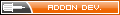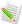Upright landers in 2016 I think there needs to be some work done for light weight landers in 2016. Not sure if it touchdown, operator related? Any thought process on how to do this?09-11-2019, 08:15 AM #2 4throck Enthusiast !This works for 2001 Aries Ib (a heavy lander) in Vessel Builder. There's minor skidding but you reach a landed state after a few second by applying brakes. The relevant parameters are: Code: ```;<-------------------------GENERAL SETTINGS - Configuration: 0-------------------------> EMPTY_MASS = 23465 VSIZE = 10 PMI = 20 20 10 CSECTIONS = 20 20 20 GRAVITYGDAMP = 0 ROTDRAG = 0.01 0.01 0.01 ..... ;<-------------------------PROPELLANT DEFINITIONS-------------------------> PRP_0_ID = 0 PRP_0_MAXMASS = 84880 PRP_0_EFFICIENCY = 1206 PRP_0_PRIMARY = TRUE PRP_0_NAME = Tank_0 .... ;<-------------------------TOUCHDOWNPOINTS DEFINITIONS-------------------------> TDP_1_0_POS = 8.66025 -5 -10 TDP_1_0_STIFFNESS = 708576 TDP_1_0_DAMPING = 498735 TDP_1_0_MU = 3 TDP_1_0_MULNG = 0.2 TDP_1_1_POS = -8.66025 -5 -10 TDP_1_1_STIFFNESS = 708576 TDP_1_1_DAMPING = 498735 TDP_1_1_MU = 3 TDP_1_1_MULNG = 0.2 TDP_1_2_POS = 0 10 -10 TDP_1_2_STIFFNESS = 708576 TDP_1_2_DAMPING = 498735 TDP_1_2_MU = 3 TDP_1_2_MULNG = 0.2 TDP_1_3_POS = 0 0 10 TDP_1_3_STIFFNESS = 708576 TDP_1_3_DAMPING = 498735 TDP_1_3_MU = 3 TDP_1_3_MULNG = 0.2``` Last edited by 4throck; 09-11-2019 at 08:17 AM.Thanked by:09-11-2019, 10:37 AM #3 gattispilot Addon DeveloperBrakes on a lander? So I try the viram lander. It was upright but then slowly moved and then ended up in space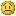Code: ```ClassName = VesselBuilder1 Module = VesselBuilder1 ;CONFIGURATION FILE FOR Viramlander NOEDITOR = FALSE CONFIGURATIONS = 1 ;<-------------------------GENERAL SETTINGS - Configuration: 0-------------------------> EMPTY_MASS = 1200 VSIZE = 10 PMI = 20 20 10 CSECTIONS = 20 20 20 GRAVITYGDAMP = 0 ROTDRAG = 0.01 0.01 0.01 ;<-------------------------MESHES DEFINITIONS-------------------------> MESH_0_NAME = vIRAMLANDER3 MESH_0_POS = 0 0 0 MESH_0_DIR = 0 0 1 MESH_0_ROT = 0 1 0 MESH_0_VIS = 1 ;<-------------------------DOCKS DEFINITIONS-------------------------> ;<-------------------------ATTACHMENTS DEFINITIONS-------------------------> ;<-------------------------ANIMATIONS DEFINITIONS-------------------------> ;<-------------------------PROPELLANT DEFINITIONS-------------------------> PRP_0_ID = 0 PRP_0_MAXMASS = 1480 PRP_0_EFFICIENCY = 1 PRP_0_PRIMARY = TRUE PRP_0_NAME = Tank_0 ;<-------------------------THRUSTERS DEFINITIONS-------------------------> ;<-------------------------THRUSTER GROUPS DEFINITIONS-------------------------> ;<-------------------------TOUCHDOWNPOINTS DEFINITIONS-------------------------> TDP_1_0_POS = 0 -1.32 5.5 TDP_1_0_STIFFNESS = 14388 TDP_1_0_DAMPING = 10127.1 TDP_1_0_MU = 0.1 TDP_1_0_MULNG = 0.2 TDP_1_1_POS = -4.33013 -1.32 -2.5 TDP_1_1_STIFFNESS = 14388 TDP_1_1_DAMPING = 10127.1 TDP_1_1_MU = 3 TDP_1_1_MULNG = 0.2 TDP_1_2_POS = 4.33013 -1.32 -2.5 TDP_1_2_STIFFNESS = 14388 TDP_1_2_DAMPING = 10127.1 TDP_1_2_MU = 3 TDP_1_2_MULNG = 0.2 TDP_1_3_POS = 0 5 0 TDP_1_3_STIFFNESS = 14388 TDP_1_3_DAMPING = 10127.1 TDP_1_3_MU = 3 TDP_1_3_MULNG = 0.2``` Viramlander:VesselBuilder1\Viramlander STATUS Orbiting Sun RPOS -142723395498.582 109304969.447 -46357522991.065 RVEL 2858104.1788 2241474.1345 -1682607.9608 AROT 104.023 26.767 134.587 VROT -14.4171 6.1901 4.9386 AFCMODE 7 PRPLEVEL 0:0.675676 NAVFREQ 0 0 END Last edited by gattispilot; 09-11-2019 at 10:48 AM.09-11-2019, 10:51 AM #4 Abdullah Radwan Addon DeveloperI use this code to stop skidding on the ground for ShuttlePB. The rotation code is from fred18. Code: ```void ShuttlePB::clbkPreStep(double simt, double simdt, double mjd) { if (!(GetFlightStatus() & 1) && GroundContact() && GetGroundspeed() <= 0.15 && !GetThrustVector(VECTOR3())) SetStatusLanded(); } void ShuttlePB::SetStatusLanded() { VESSELSTATUS2 status; memset(&status, 0, sizeof(status)); status.version = 2; GetStatusEx(&status); status.status = 1; MATRIX3 rot1 = RotationMatrix({ 0 * RAD, 90 * RAD - status.surf_lng, 0 * RAD }); MATRIX3 rot2 = RotationMatrix({ -status.surf_lat + 0 * RAD, 0, 0 * RAD }); MATRIX3 rot3 = RotationMatrix({ 0, 0, 180 * RAD + status.surf_hdg }); MATRIX3 rot4 = RotationMatrix({ 90 * RAD, 0, 0 }); MATRIX3 RotMatrix_Def = mul(rot1, mul(rot2, mul(rot3, rot4))); status.arot.x = atan2(RotMatrix_Def.m23, RotMatrix_Def.m33); status.arot.y = -asin(RotMatrix_Def.m13); status.arot.z = atan2(RotMatrix_Def.m12, RotMatrix_Def.m11); status.vrot.x = 1.1; // The lander elevation from the ground DefSetStateEx(&status); } MATRIX3 ShuttlePB::RotationMatrix(VECTOR3 angles) { MATRIX3 m; MATRIX3 RM_X, RM_Y, RM_Z; RM_X = _M(1, 0, 0, 0, cos(angles.x), -sin(angles.x), 0, sin(angles.x), cos(angles.x)); RM_Y = _M(cos(angles.y), 0, sin(angles.y), 0, 1, 0, -sin(angles.y), 0, cos(angles.y)); RM_Z = _M(cos(angles.z), -sin(angles.z), 0, sin(angles.z), cos(angles.z), 0, 0, 0, 1); m = mul(RM_X, mul(RM_Y, RM_Z)); return m; }```Thanked by:09-11-2019, 11:56 AM #5 gattispilot Addon DeveloperThanks. Those that can code can add that. But that doesn't address the issue of why that occurs. I guess if it spins enough it ends up in orbit?09-11-2019, 12:57 PM #6 Abdullah Radwan Addon DeveloperQuote: Originally Posted by gattispilot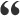Thanks. Those that can code can add that. But that doesn't address the issue of why that occurs. I guess if it spins enough it ends up in orbit? If you're asking about the skidding, it's a bug in Orbiter 2016 core. Have you tried Fred18 touchdown code if you have problems with the touchdown points?09-11-2019, 02:13 PM #7 gattispilot Addon DeveloperOh. Yes I have used Fred's code. But it spins in VB. But like you said it is a bug on the core09-11-2019, 03:01 PM #8 4throck Enthusiast !Quote: Originally Posted by gattispilotBrakes on a lander? YesSome parameters (friction?) only have effect when you apply brakes. This is to allow for driving on the surface (like the DG) with brakes off. So right after landing you apply brakes. The residual acceleration dampens goes to zero in a few seconds, and your vessel goes into a landed state. Of course, if you land on a steep slope, your brake strength is not hold your vessel in place. In that case skidding or tumbling is normal. That's how it works for the heavy lander example I posted. Last edited by 4throck; 09-11-2019 at 03:08 PM.09-11-2019, 03:07 PM #9 gattispilot Addon DeveloperOh. So what if we just increase the surface friction to a high number. So brake will be simulated?09-11-2019, 07:28 PM #10 4throck Enthusiast !Brakes are separate from landing points and strength is in Newtons.09-11-2019, 07:34 PM #11 gattispilot Addon DeveloperSo i was looking for brakes in the VB doc but didn't see it. Might have an older version09-11-2019, 07:47 PM #12 4throck Enthusiast !Just to be clear, I was wrong here: "Some parameters (friction?) only have effect when you apply brakes." Brakes are separate from landing points and don't affect them. ---------- Post added at 20:47 ---------- Previous post was at 20:45 ---------- Quote: Originally Posted by gattispilotSo i was looking for brakes in the VB doc but didn't see it. Might have an older version Don't think Fred documented it, If I'm not mistaken it was one of the last things he added. You have info about brakes on the SDK docs. Check page 414 of the API_Reference.pdf ---------------------------------- Here's a working VB light lander, 2000kg including fuel.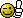Code: ```ClassName = VesselBuilder1 Module = VesselBuilder1 ;CONFIGURATION FILE FOR light lander NOEDITOR = FALSE CONFIGURATIONS = 1 ;<-------------------------GENERAL SETTINGS - Configuration: 0-------------------------> EMPTY_MASS = 1000 VSIZE = 10 PMI = 20 20 10 CSECTIONS = 20 20 20 GRAVITYGDAMP = 0 ROTDRAG = 0.01 0.01 0.01 ;<-------------------------MESHES DEFINITIONS-------------------------> MESH_0_NAME = Carina MESH_0_POS = 0 0 0.9 MESH_0_DIR = 0 0 1 MESH_0_ROT = 0 1 0 MESH_0_VIS = 1 ;<-------------------------DOCKS DEFINITIONS-------------------------> ;<-------------------------ATTACHMENTS DEFINITIONS-------------------------> ;<-------------------------ANIMATIONS DEFINITIONS-------------------------> ;<-------------------------PROPELLANT DEFINITIONS-------------------------> PRP_0_ID = 0 PRP_0_MAXMASS = 1000 PRP_0_EFFICIENCY = 1 PRP_0_PRIMARY = TRUE PRP_0_NAME = Tank_0 ;<-------------------------THRUSTERS DEFINITIONS-------------------------> THR_0_ID = 0 THR_0_NAME = Pitch_up_1 THR_0_POS = 0 0 10 THR_0_DIR = 0 1 0 THR_0_MAX0 = 104.72 THR_0_ISP0 = 1e+007 THR_0_ISPREF = 0 THR_0_PREF = 101400 THR_0_TANK = 0 THR_0_HASEXHAUST = FALSE THR_0_HASPARTICLES = FALSE THR_1_ID = 1 THR_1_NAME = Pitch_up_2 THR_1_POS = 0 0 -10 THR_1_DIR = 0 -1 0 THR_1_MAX0 = 104.72 THR_1_ISP0 = 1e+007 THR_1_ISPREF = 0 THR_1_PREF = 101400 THR_1_TANK = 0 THR_1_HASEXHAUST = FALSE THR_1_HASPARTICLES = FALSE THR_2_ID = 2 THR_2_NAME = Pitch_dwn_1 THR_2_POS = 0 0 10 THR_2_DIR = 0 -1 0 THR_2_MAX0 = 104.72 THR_2_ISP0 = 1e+007 THR_2_ISPREF = 0 THR_2_PREF = 101400 THR_2_TANK = 0 THR_2_HASEXHAUST = FALSE THR_2_HASPARTICLES = FALSE THR_3_ID = 3 THR_3_NAME = Pitch_dwn_2 THR_3_POS = 0 0 -10 THR_3_DIR = 0 1 0 THR_3_MAX0 = 104.72 THR_3_ISP0 = 1e+007 THR_3_ISPREF = 0 THR_3_PREF = 101400 THR_3_TANK = 0 THR_3_HASEXHAUST = FALSE THR_3_HASPARTICLES = FALSE THR_4_ID = 4 THR_4_NAME = Yaw_left_1 THR_4_POS = 0 0 10 THR_4_DIR = -1 0 0 THR_4_MAX0 = 104.72 THR_4_ISP0 = 1e+007 THR_4_ISPREF = 0 THR_4_PREF = 101400 THR_4_TANK = 0 THR_4_HASEXHAUST = FALSE THR_4_HASPARTICLES = FALSE THR_5_ID = 5 THR_5_NAME = Yaw_left_2 THR_5_POS = 0 0 -10 THR_5_DIR = 1 0 0 THR_5_MAX0 = 104.72 THR_5_ISP0 = 1e+007 THR_5_ISPREF = 0 THR_5_PREF = 101400 THR_5_TANK = 0 THR_5_HASEXHAUST = FALSE THR_5_HASPARTICLES = FALSE THR_6_ID = 6 THR_6_NAME = Yaw_right_1 THR_6_POS = 0 0 10 THR_6_DIR = 1 0 0 THR_6_MAX0 = 104.72 THR_6_ISP0 = 1e+007 THR_6_ISPREF = 0 THR_6_PREF = 101400 THR_6_TANK = 0 THR_6_HASEXHAUST = FALSE THR_6_HASPARTICLES = FALSE THR_7_ID = 7 THR_7_NAME = Yaw_right_2 THR_7_POS = 0 0 -10 THR_7_DIR = -1 0 0 THR_7_MAX0 = 104.72 THR_7_ISP0 = 1e+007 THR_7_ISPREF = 0 THR_7_PREF = 101400 THR_7_TANK = 0 THR_7_HASEXHAUST = FALSE THR_7_HASPARTICLES = FALSE THR_8_ID = 8 THR_8_NAME = Bank_left_1 THR_8_POS = 10 0 0 THR_8_DIR = 0 1 0 THR_8_MAX0 = 52.3599 THR_8_ISP0 = 1e+007 THR_8_ISPREF = 0 THR_8_PREF = 101400 THR_8_TANK = 0 THR_8_HASEXHAUST = FALSE THR_8_HASPARTICLES = FALSE THR_9_ID = 9 THR_9_NAME = Bank_left_2 THR_9_POS = -10 0 0 THR_9_DIR = 0 -1 0 THR_9_MAX0 = 52.3599 THR_9_ISP0 = 1e+007 THR_9_ISPREF = 0 THR_9_PREF = 101400 THR_9_TANK = 0 THR_9_HASEXHAUST = FALSE THR_9_HASPARTICLES = FALSE THR_10_ID = 10 THR_10_NAME = Bank_right_1 THR_10_POS = 10 0 0 THR_10_DIR = 0 -1 0 THR_10_MAX0 = 52.3599 THR_10_ISP0 = 1e+007 THR_10_ISPREF = 0 THR_10_PREF = 101400 THR_10_TANK = 0 THR_10_HASEXHAUST = FALSE THR_10_HASPARTICLES = FALSE THR_11_ID = 11 THR_11_NAME = Bank_right_2 THR_11_POS = -10 0 0 THR_11_DIR = 0 1 0 THR_11_MAX0 = 52.3599 THR_11_ISP0 = 1e+007 THR_11_ISPREF = 0 THR_11_PREF = 101400 THR_11_TANK = 0 THR_11_HASEXHAUST = FALSE THR_11_HASPARTICLES = FALSE THR_12_ID = 12 THR_12_NAME = Translate_up THR_12_POS = 0 0 0 THR_12_DIR = 0 1 0 THR_12_MAX0 = 300 THR_12_ISP0 = 1e+007 THR_12_ISPREF = 0 THR_12_PREF = 101400 THR_12_TANK = 0 THR_12_HASEXHAUST = FALSE THR_12_HASPARTICLES = FALSE THR_13_ID = 13 THR_13_NAME = Translate_dwn THR_13_POS = 0 0 0 THR_13_DIR = 0 -1 0 THR_13_MAX0 = 300 THR_13_ISP0 = 1e+007 THR_13_ISPREF = 0 THR_13_PREF = 101400 THR_13_TANK = 0 THR_13_HASEXHAUST = FALSE THR_13_HASPARTICLES = FALSE THR_14_ID = 14 THR_14_NAME = Translate_left THR_14_POS = 0 0 0 THR_14_DIR = -1 0 0 THR_14_MAX0 = 300 THR_14_ISP0 = 1e+007 THR_14_ISPREF = 0 THR_14_PREF = 101400 THR_14_TANK = 0 THR_14_HASEXHAUST = FALSE THR_14_HASPARTICLES = FALSE THR_15_ID = 15 THR_15_NAME = Translate_right THR_15_POS = 0 0 0 THR_15_DIR = 1 0 0 THR_15_MAX0 = 300 THR_15_ISP0 = 1e+007 THR_15_ISPREF = 0 THR_15_PREF = 101400 THR_15_TANK = 0 THR_15_HASEXHAUST = FALSE THR_15_HASPARTICLES = FALSE THR_16_ID = 16 THR_16_NAME = Translate_forward THR_16_POS = 0 0 0 THR_16_DIR = 0 0 1 THR_16_MAX0 = 30000 THR_16_ISP0 = 1e+007 THR_16_ISPREF = 0 THR_16_PREF = 101400 THR_16_TANK = 0 THR_16_HASEXHAUST = FALSE THR_16_HASPARTICLES = FALSE THR_17_ID = 17 THR_17_NAME = Translate_backward THR_17_POS = 0 0 0 THR_17_DIR = 0 0 -1 THR_17_MAX0 = 300 THR_17_ISP0 = 1e+007 THR_17_ISPREF = 0 THR_17_PREF = 101400 THR_17_TANK = 0 THR_17_HASEXHAUST = FALSE THR_17_HASPARTICLES = FALSE ;<-------------------------THRUSTER GROUPS DEFINITIONS-------------------------> THGROUP_MAIN = TRUE THGROUP_MAIN_THRUSTERS = 16 THGROUP_RETRO = TRUE THGROUP_RETRO_THRUSTERS = 17 THGROUP_HOVER = TRUE THGROUP_HOVER_THRUSTERS = 12 THGROUP_ATT_PITCHUP = TRUE THGROUP_ATT_PITCHUP_THRUSTERS = 0, 1 THGROUP_ATT_PITCHDOWN = TRUE THGROUP_ATT_PITCHDOWN_THRUSTERS = 2, 3 THGROUP_ATT_YAWLEFT = TRUE THGROUP_ATT_YAWLEFT_THRUSTERS = 4, 5 THGROUP_ATT_YAWRIGHT = TRUE THGROUP_ATT_YAWRIGHT_THRUSTERS = 6, 7 THGROUP_ATT_BANKLEFT = TRUE THGROUP_ATT_BANKLEFT_THRUSTERS = 8, 9 THGROUP_ATT_BANKRIGHT = TRUE THGROUP_ATT_BANKRIGHT_THRUSTERS = 10, 11 THGROUP_ATT_RIGHT = TRUE THGROUP_ATT_RIGHT_THRUSTERS = 15 THGROUP_ATT_LEFT = TRUE THGROUP_ATT_LEFT_THRUSTERS = 14 THGROUP_ATT_UP = TRUE THGROUP_ATT_UP_THRUSTERS = 12 THGROUP_ATT_DOWN = TRUE THGROUP_ATT_DOWN_THRUSTERS = 13 THGROUP_ATT_FORWARD = TRUE THGROUP_ATT_FORWARD_THRUSTERS = 16 THGROUP_ATT_BACK = TRUE THGROUP_ATT_BACK_THRUSTERS = 17 ;<-------------------------TOUCHDOWNPOINTS DEFINITIONS-------------------------> TDP_1_0_POS = 1.73205 -1 -0.1 TDP_1_0_STIFFNESS = 13080 TDP_1_0_DAMPING = 9206.43 TDP_1_0_MU = 3 TDP_1_0_MULNG = 0.2 TDP_1_1_POS = -1.73205 -1 -0.1 TDP_1_1_STIFFNESS = 13080 TDP_1_1_DAMPING = 9206.43 TDP_1_1_MU = 3 TDP_1_1_MULNG = 0.2 TDP_1_2_POS = 0 2 -0.1 TDP_1_2_STIFFNESS = 13080 TDP_1_2_DAMPING = 9206.43 TDP_1_2_MU = 3 TDP_1_2_MULNG = 0.2 TDP_1_3_POS = 0 0 2 TDP_1_3_STIFFNESS = 13080 TDP_1_3_DAMPING = 9206.43 TDP_1_3_MU = 3 TDP_1_3_MULNG = 0.2 ;<-------------------------AIRFOILS DEFINITIONS-------------------------> ;<-------------------------CONTROL SURFACES DEFINITIONS-------------------------> ;<-------------------------CAMERA DEFINITIONS-------------------------> ;<-------------------------VIRTUAL COCKPIT DEFINITIONS-------------------------> ;<-------------------------LIGHTS: BEACONS DEFINITIONS-------------------------> ;<-------------------------LIGHTS: LIGHT EMITTERS DEFINITIONS-------------------------> ;<-------------------------VARIABLE DRAG ITEMS DEFINITIONS-------------------------> ;<-------------------------EVENTS DEFINITIONS-------------------------> ;<-------------------------EXHAUST TEXTURES DEFINITIONS-------------------------> ;<-------------------------PARTICLES DEFINITIONS------------------------->``` Last edited by 4throck; 09-12-2019 at 12:03 AM.Thanked by:09-12-2019, 09:22 AM #13 gattispilot Addon DeveloperI didn't see a brake setting in that cfg? I fired up my lander and although it didn't lift off it move and then stop. no spinning Which is the latest VB? Last edited by gattispilot; 09-12-2019 at 12:32 PM.09-12-2019, 04:39 PM #14 4throck Enthusiast !The latest version is "VesselBuilder1_PreRelease". There's no specific parameter for brakes I think. But it's discussed here (with an update): https://www.orbiter-forum.com/showth...&postcount=351Thanked by:

 Thread ToolsShow Printable VersionEmail this PagePosting Rules BB code is On Smilies are On [IMG] code is On HTML code is Off You may not post new threads You may not post replies You may not post attachments You may not edit your posts
 Forum Jump User Control Panel Private Messages Subscriptions Who's Online Search Forums Forums Home Orbiter-Forum.com     Announcements     Meets & Greets Orbiter Space Flight Simulator     Orbiter Web Forum         OFMM         Orbiter Forum Space Station         Simpit Forum     General Questions & Help     MFD Questions & Help     Hardware & Software Help     Tutorials & Challenges     Orbiter SDK     Orbiter Visualization Project     Orbiter Beta » Orbiter Project Orbiter Addons     OrbitHangar Addons & Comments     Addons     Addon Development     Addon Requests     Addon Support & Bugs         Addon Developer Forums             Project Apollo - NASSP     Orbiter Lua Scripting Far Side of the Moon     Spaceflight News     Math & Physics     Astronomy & the Night Sky     Backyard Rocketry     Brighton Lounge     International Forum

All times are GMT. The time now is 11:25 AM.